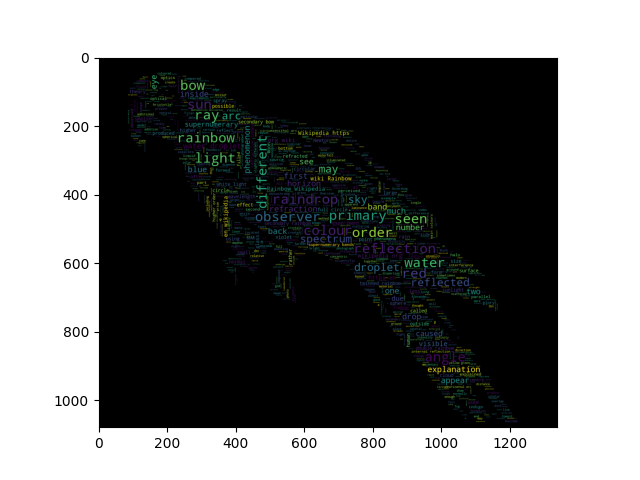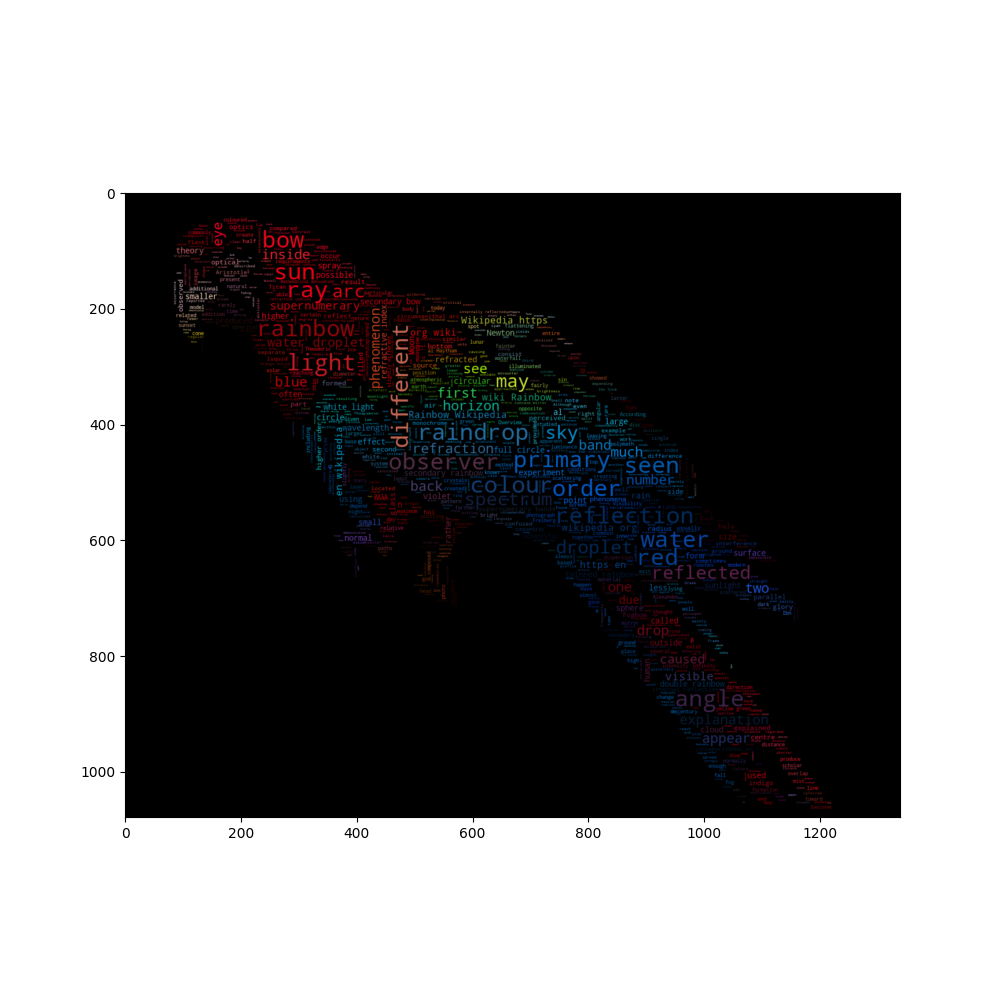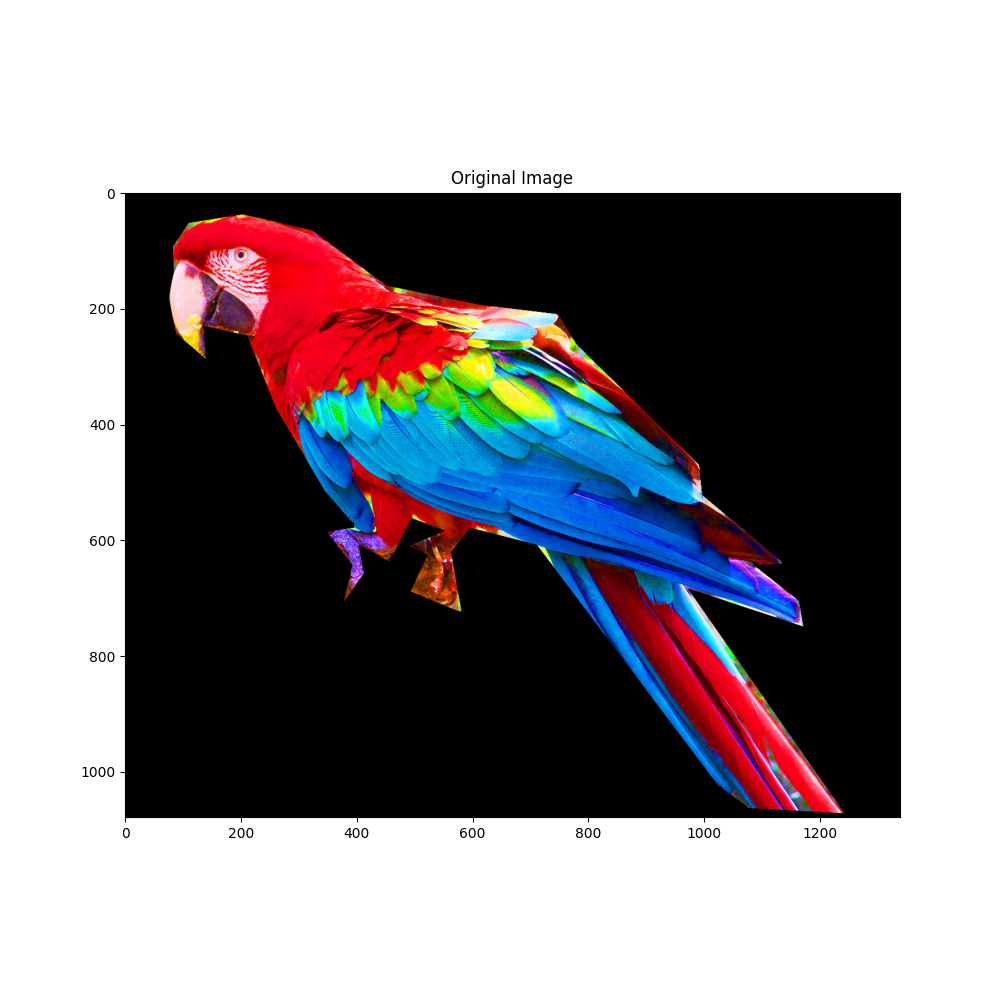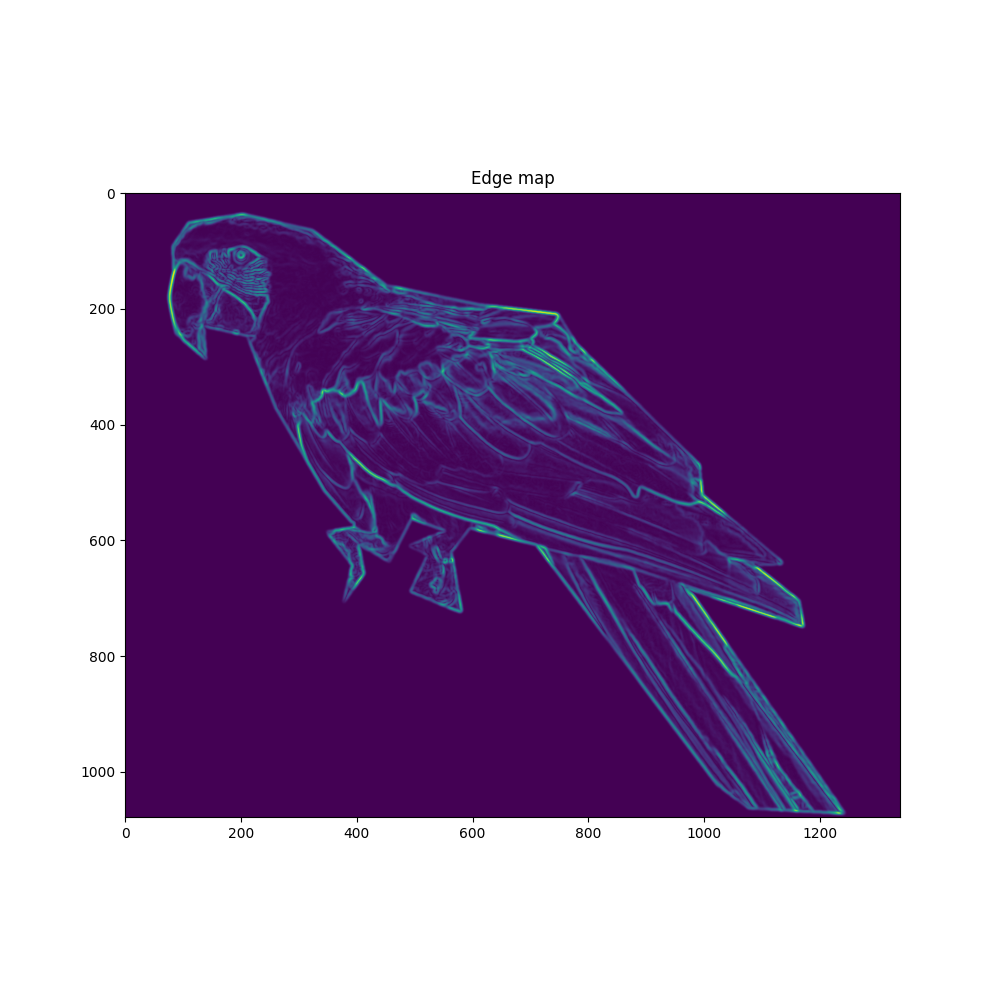# Image-colored wordcloud with boundary map¶

A slightly more elaborate version of an image-colored wordcloud that also takes edges in the image into account. Recreating an image similar to the parrot example.

••••```import os
from PIL import Image

import numpy as np
import matplotlib.pyplot as plt

from wordcloud import WordCloud, ImageColorGenerator

# get data directory (using getcwd() is needed to support running example in generated IPython notebook)
d = os.path.dirname(__file__) if "__file__" in locals() else os.getcwd()

# load wikipedia text on rainbow

# load image. This has been modified in gimp to be brighter and have more saturation.
parrot_color = np.array(Image.open(os.path.join(d, "parrot-by-jose-mari-gimenez2.jpg")))
# subsample by factor of 3. Very lossy but for a wordcloud we don't really care.
parrot_color = parrot_color[::3, ::3]

# some finesse: we enforce boundaries between colors so they get less washed out.
# For that we do some edge detection in the image
edges = np.mean([gaussian_gradient_magnitude(parrot_color[:, :, i] / 255., 2) for i in range(3)], axis=0)

# create wordcloud. A bit sluggish, you can subsample more strongly for quicker rendering
# relative_scaling=0 means the frequencies in the data are reflected less
# acurately but it makes a better picture

# generate word cloud
wc.generate(text)
plt.imshow(wc)

# create coloring from image
image_colors = ImageColorGenerator(parrot_color)
wc.recolor(color_func=image_colors)
plt.figure(figsize=(10, 10))
plt.imshow(wc, interpolation="bilinear")
wc.to_file("parrot_new.png")

plt.figure(figsize=(10, 10))
plt.title("Original Image")
plt.imshow(parrot_color)

plt.figure(figsize=(10, 10))
plt.title("Edge map")
plt.imshow(edges)
plt.show()
```

Total running time of the script: ( 0 minutes 21.403 seconds)

Gallery generated by Sphinx-Gallery